### Home > A2C > Chapter Ch11 > Lesson 11.3.2 > Problem11-149

11-149.
1. Solve and check each equation. Homework Help ✎

1.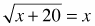2.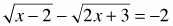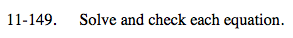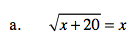Square each side.
Set the equation equal to zero
Factor the equation.

x + 20 = x2
0 = x2x − 20

x = 5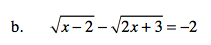$\sqrt{x-2}+2=\sqrt{2x+3}$

$x-2+2\sqrt{x-2}+4=2x+3$

Here is the start of the process.
Complete the rest and check all possible answers.

x = 3 x = 11